﻿ 非冻结可破碎模型冰的实验设计与分析

# 非冻结可破碎模型冰的实验设计与分析Experimental Design and Analysis of Breakable Artificial Model Ice

Abstract: According to the type of model ice, the ship-ice model test can be divided into frozen model ice test in ice tank and artificial model ice test in conventional towing tank. In this paper, a new type of ar-tificial model ice is developed on the basis of summarizing the characteristics of the model ice in ice tank and the model rate of the ship-ice model test. Uniaxial compression and three point bending tests were performed on the artificial model ice according to the test method recommended by the International Towing Tank Conference. The results show that the artificial model ice can be used for ship-ice interaction test at a scale ratio of 1:30.

1. 引言

2. 实验原理

Table 1. Summary of scaling laws according to Froude and Cauchy scaling (Timco, 1984)

${\sigma }_{c}=\frac{F}{ci*mkbh}$ (1)

${\sigma }_{f}=\frac{F}{W}=\frac{3FL}{2b{h}^{2}}$ (2)

3. 实验设计

3.1. 实验材料与仪器

3.2. 试样制备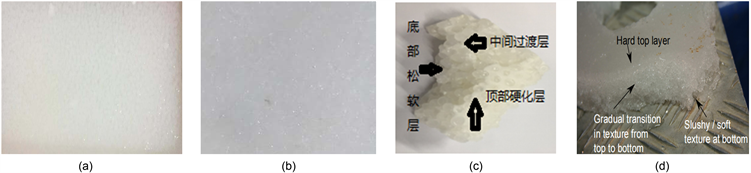Figure 1. Preparation of crushed artificialmodel Ice model ice specimens

3.3. 试件强度测定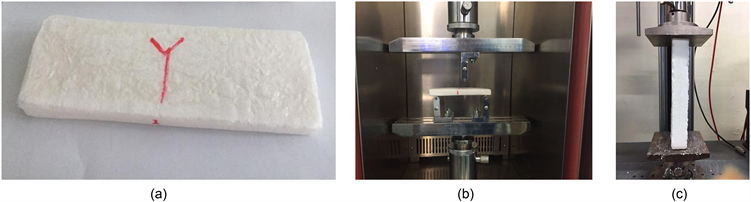Figure 2. Mechanical properties test of artificialmodel Ice, model ice Specimen (a), three-point bending test (b), uniaxial compression test (c)

3.4. 试验结果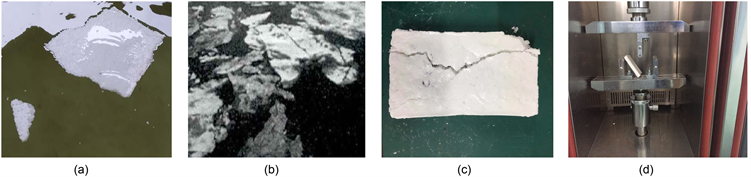Figure 3. Results of non-frozen model ice, comparison of natural sea ice floating state (a), (b), uniaxial compression test (c), three-point bending test (d)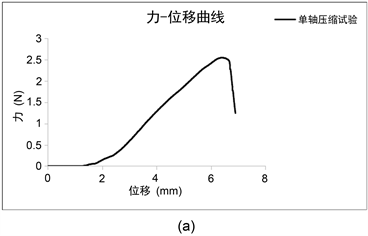Figure 4. Uniaxial compression test and three point bending test results, force-displacement curves

4. 分析与讨论

4.1. 不同比例对材料的影响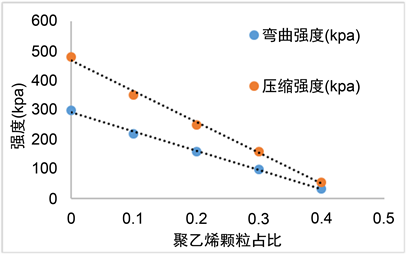Figure 5. Curve: different ice strength curves of the model with different ratio

4.2. 模型冰的力学特征分析

5. 总结

NOTES

*通讯作者。

 李振福, 孙悦, 韦博文. “冰上丝绸之路”——北极航线船舶航行安全的跟驰模型[J]. 大连海事大学学报, 2018, 44(3): 22-27.

 王梦颖, 穆顷, 张晓频, 张霖, 李明. 渤海固定结构物冰力特点及计算方法[J]. 船海工程, 2016, 45(5): 135-138.

 王超, 叶礼裕, 常欣, 李兴. 非接触工况下冰桨干扰水动力载荷试验[J]. 哈尔滨工程大学学报, 2017, 38(8): 1190-1196.

 黄焱, 马高强, 孙剑桥. 船–冰碰撞载荷时间历程的模型试验研究[J]. 振动与冲击, 2019, 38(4): 7-14.

 郭春雨, 徐佩, 张海鹏. 冰对螺旋桨水动力性能影响的试验研究[J]. 船海力学, 2018, 22(7): 797-806.

 康瑞. 平整冰中破冰船操纵性能预报[D]: [硕士学位论文]. 哈尔滨: 哈尔滨工程大学, 2016.

 Huang, Y., Huang, S.Y., et al. (2018) Experiments on Navigating Resistance of an Icebreaker in Snow Covered Level Ice. Cold Regions Science and Technology, 152, 1-14.
https://doi.org/10.1016/j.coldregions.2018.04.007

 王庆凯. 北极航道融冰期海冰物理和力学工程参数研究[D]: [博士学位论文]. 大连: 大连理工大学, 2019.

 Kim, H. and Colbourne, B. (2016) A Study of the Effect of Contact Surface Geometry and Crushing Rate on the Behavior of the Ice. Ocean Engineering, 126, 240-253.
https://doi.org/10.1016/j.oceaneng.2016.09.025

 Jeong, S.-Y., et al. (2017) Implementation of Ship Perfor-mance Test in Brash Ice Channel. Ocean Engineering, 140, 57-65.
https://doi.org/10.1016/j.oceaneng.2017.05.008

 Luo, W.-Z., Guo, C.-Y., et al. (2018) Experimental Research on Resistance and Motion Attitude Variation of Ship- Wave-Ice Interaction in Marginal Ice Zones. Marine Structures, 58, 399-415.
https://doi.org/10.1016/j.marstruc.2017.12.013

 Kvadsheim, S. (2014) Ice Ridge Keel Size Distributions: Analysis of Ice Ridge Keel Thickness from Upward Looking Sonar Data from the Fram Strait Using Extreme Value Theory. NTNU.

 Zhang, J., et al. (2019) A Stochastic Method for the Prediction of Icebreaker Bow Extreme Stresses. Applied Ocean Research, 87, 95-101.
https://doi.org/10.1016/j.apor.2019.03.019

Top